Courses

# Transfer Function

## 10 Questions MCQ Test Topicwise Question Bank for GATE Electrical Engineering | Transfer Function

Description
This mock test of Transfer Function for Electronics and Communication Engineering (ECE) helps you for every Electronics and Communication Engineering (ECE) entrance exam. This contains 10 Multiple Choice Questions for Electronics and Communication Engineering (ECE) Transfer Function (mcq) to study with solutions a complete question bank. The solved questions answers in this Transfer Function quiz give you a good mix of easy questions and tough questions. Electronics and Communication Engineering (ECE) students definitely take this Transfer Function exercise for a better result in the exam. You can find other Transfer Function extra questions, long questions & short questions for Electronics and Communication Engineering (ECE) on EduRev as well by searching above.
*Multiple options can be correct
QUESTION: 1

### Non-minimum phase transfer function is defined as the transfer function

Solution:

The non-minimum phase transfer function is defined as the transfer function is defined as the transfer function which has zeros (or) poles in right side of s-plane

QUESTION: 2

### The type and order of the system described by the open loop transfer function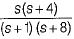are respectively

Solution: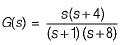There is no open loop pole at origin. Hence, type of system = 0.
Highest power of s of the characteristic equation 1+ G(s) = 0 will be 2.
Hence, order of given System = 2.

QUESTION: 3

### Assertion (A): If the number of zeros are less than the number of poles (i.e. Z < P), we say that there are zeros at infinity and the order of such zeros is P-Z Reason (R): The value of the transfer function becomes zero for s tends to zero.

Solution:

For Z < P,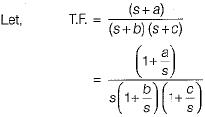∴ Here, number of zeros = 1 and no. of poles = 2
∴ P - Z = 1
When s →∞, transfer function becomes zero. Thus, there is one zero (P - Z = 1) at infinity. Thus, assertion is true.
Since value of transfer function becomes zero as s →∞ therefore, reason is false.

QUESTION: 4

The transfer function ofthe network shown below is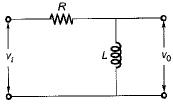Solution:

Let i be the current in the given circuit.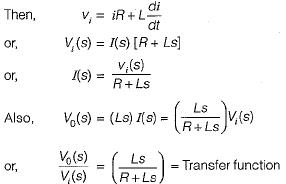QUESTION: 5

Consider the following statements regarding the properties of transfer function:
1. The transfer function is independent of the input of the system.
2. The transfer function is defined for linear time- variant system.
3. The transfer function of a continuous-data system is expressed only as a function of the complex variable s.
4. All the initial conditions are not necessarily set to zero for finding the transfer function of a given system.

Q. Which of the above statements is/are not true?

Solution:

Explanation : The properties of transfer function are given below:

1) The ratio of Laplace transform of output to Laplace transform of input assuming all initial conditions to be zero.

2) The transfer function of a system is the Laplace transform of its impulse response under assumption of zero initial conditions.

3) Replacing ‘s’ variable with linear operation D = d/dt in transfer function of a system, the differential equation of the system can be obtained.

4) The transfer function of a system does not depend on the inputs to the system.

5) The system poles and zeros can be determined from its transfer function.

QUESTION: 6

​The differential equation of a control system having input x(t) and output y(t) is given as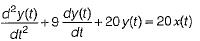The output response of the system for unit step input is given by

Solution:

Given differential equation is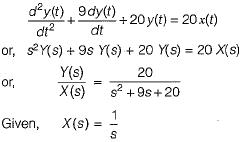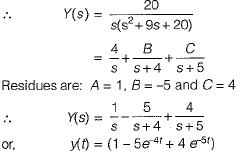QUESTION: 7

The step response of a system is given by
c(t) = 1 + 0.25 e-50t - 1.25e-10t
The steady state gain of the transfer function in time constant form will be

Solution:

Given, c(t) = 1 + 0.25 e-50t - 1.25e-10t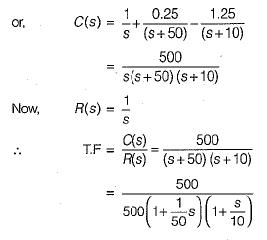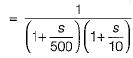Thus,C(s)/R(s) is in time constant form having steady state gain = 1.

QUESTION: 8

The poles and zeros of the transfer function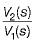for the network shown below are located at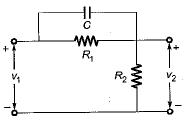Solution:

For given network, total input impedance is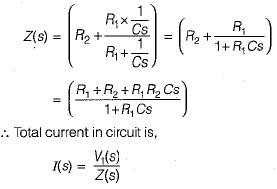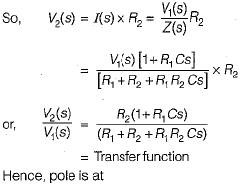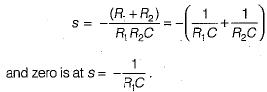QUESTION: 9

The pole-zero configuration of a transfer function is shown below: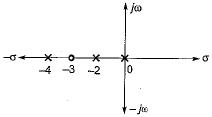If the value of the transfer function at s = 1 is 3.2, the gain factor K is

Solution:

From given pole-zero plot, transfer function is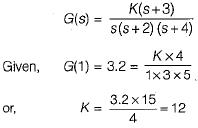So, gain factor K =12

QUESTION: 10

The type of a system denotes the number of

Solution:

The type of a system denotes the no. of poles of origin.